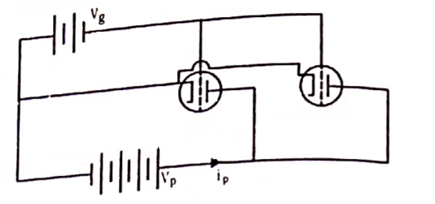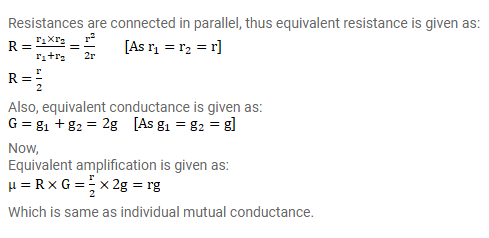# Figure shows two identical triode tubes connected in parallel.

Question:

Figure shows two identical triode tubes connected in parallel. The anodes are connected together, the grids are connected together. Show that the equivalent plate resistance is half of the individual plate resistance, the equivalent mutual conductance is double the individual conductance and the equivalent amplification factor is the same as the individual amplification factor.Solution: